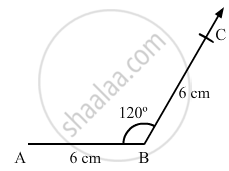Share

# Construct a Quadrilateral Abcd in Which Ab = Bc = 6 Cm, Ad = Dc = 4.5 Cm and ∠B = 120°. - Mathematics

Course
ConceptConstructing a Quadrilateral When Two Adjacent Sides and Three Angles Are Known

#### Question

Construct a quadrilateral ABCD in which AB = BC = 6 cm, AD = DC = 4.5 cm and ∠B = 120°.

#### Solution

Steps of construction:

Step I: Draw AB = 6 cm .

Step II: Construct ABC = 120°.

Step III : With B as the centre and radius 6 cm, cut off BC = 6 cm .

Now, we can see that AC is about 10 . 3 cm which is greater than AD + CD = 4 . 5 + 4 . 5 = 9 cm .

We know that sum of the lengths of two sides of triangle is always greater than the third side but here, the sum of AD and CD is less than AC .

So, construction of the given quadrilateral is not possible .Is there an error in this question or solution?

#### APPEARS IN

RD Sharma Solution for Mathematics for Class 8 by R D Sharma (2019-2020 Session) (2017 to Current)
Chapter 18: Practical Geometry (Constructions)
Ex. 18.3 | Q: 6 | Page no. 8
Solution Construct a Quadrilateral Abcd in Which Ab = Bc = 6 Cm, Ad = Dc = 4.5 Cm and ∠B = 120°. Concept: Constructing a Quadrilateral - When Two Adjacent Sides and Three Angles Are Known.
S